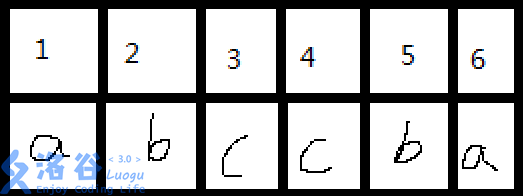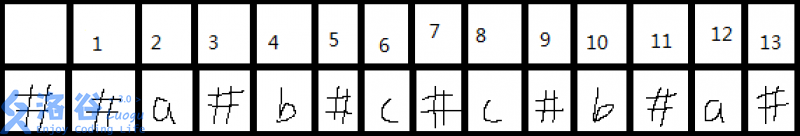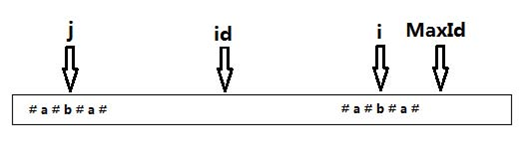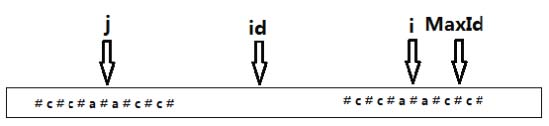# 这里将讲述  字符串算法2：Manacher算法

### 问题：给出字符串S(限制见后)求出最大回文子串长度【图是哪里偷过来的，足以说明问题】

------------------（正文分割线）----------------------

#### 重点来了：

P[i]-1代表什么？ 原串（不插入#前）中第i位置的回文串长度（包含中间点）

1、显然L=2*P[i]-1即为新串（加#）中以第i个点为中心最长回文串长度。
2、以第i位 为中心的回文串一定是以#开头和#结尾的，例如“#b#b#”或“#b#a#b#”

【再偷2张图片】maxId表示前面运行过程中求得最大回文串的最右端

id（后面程序是MID）表示此时最大回文串的对称点，i代表当前遍历到第i个位置

manacher算法只需要线性扫描一遍预处理后的字符串。

1. (i<MaxId)

•      Maxid-i>p[j] p[j]=p[i]
•      其他情况 p[i]=MaxId-i

2.其他情况 p[i]=0

1. 在i<MaxId的情况下，p的值可以在O(1) 时间内确定
2. 在i>MaxId 的情况下，p的值需要O(n) 的时间内确定，

1.while()循环本身的时间复杂度在没有前提条件的情况下确实是O(n)

2.但是这里的MaxId，是不断往后走而不可能往前退的，它自身的值的变化是递增的。

i 的值必然是比MaxId大的，

while循环执行的操作数为n次（线性次），

贴下代码：

# include <bits/stdc++.h>
using namespace std;
const int MAXN=11000005*2;
char a[2*MAXN];
int p[2*MAXN];
int main()
{
char ch=getchar();int t=0; a='?';//为了保险起见a和最后的符号不能一样
while (isalpha(ch)) {
t++;
a[2*t]=ch; a[2*t-1]='#';
ch=getchar();
}
a[2*t+1]='#';
int n=2*t+1;
int MID=0,R=0,i; //MID就是id，R就是MaxId，i就是i
for (i=1;i<=n;i++) {
if (R>i) p[i]=min(p[2*MID-i],R-i);
else p[i]=1;
while (a[i-p[i]]==a[i+p[i]]) p[i]++;
if (i+p[i]>R) R=i+p[i],MID=i;
}
int ans=0;
for (int i=1;i<=n;i++) ans=max(ans,p[i]-1);
printf("%d\n",ans);
return 0;
}

### P3805 【模板】manacher算法

#### 输入输出样例

aaa

3

#### 说明

这里有着注意点：不能判断回车否则是TLE，应该判断不是字母时果断跳出

posted @ 2018-08-28 14:31  ljc20020730  阅读(129)  评论(0编辑  收藏  举报